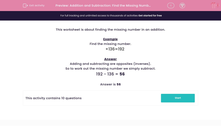# Addition and Subtraction: Find the Missing Number (1)

In this worksheet, students use subtraction to find the missing number in an addition.This content is premium and exclusive to EdPlace subscribers.Key stage:  KS 2

Curriculum topic:   Maths and Numerical Reasoning

Curriculum subtopic:   Mixed Problems

Difficulty level:#### Worksheet Overview

Example

Find the missing number.

+ 136 = 192

Adding and subtracting are opposites (inverses).

So to work out the missing number we simply subtract.

192 - 136 = 56